### Showing videos fromEddie Woo with a total of 4,314 videos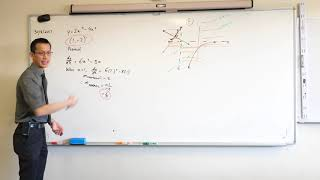05:11
Finding the equation of a normal at a given point
2y8m ago06:13
Solving an Inequality with a Rational Function (2 of 2: Solving algebraically)
2y8m ago10:36
Solving an Inequality with a Rational Function (1 of 2: Solving graphically)
2y8m ago08:13
Trigonometric Ratios of Complementary Angles (3 of 3: Constructing a helpful triangle)
2y8m ago05:45
Trigonometric Ratios of Complementary Angles (2 of 3: Basic questions)
2y8m ago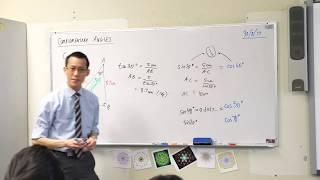08:29
Trigonometric Ratios of Complementary Angles (1 of 3: Noticing the pattern)
2y8m ago10:05
Interpreting Linear Functions (2 of 2: Example questions)
2y8m ago11:35
Interpreting Linear Functions (1 of 2: Identifying the components of an equation)
2y8m ago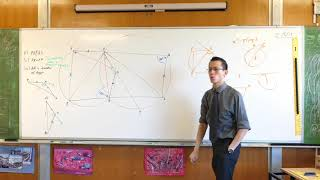06:54
Tough Circle Geometry Question (3 of 3: Using tangent properties)
2y8m ago07:48
Tough Circle Geometry Question (2 of 3: Proving a given chord is a diameter)
2y8m ago11:53
Tough Circle Geometry Question (1 of 3: Finding & using congruent triangles)
2y8m ago08:19
Differentiating a Rational Function by First Principles
2y8m ago09:40
Piecemeal Functions (1 of 2: Constructing the graph)
2y8m ago04:26
Translations of the Parabola (2 of 2: Updated standard forms)
2y8m ago14:40
Translations of the Parabola (1 of 2: Finding the equation from its features)
2y8m ago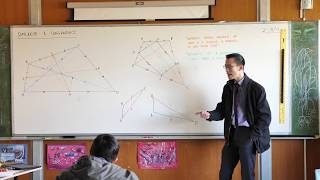07:08
Midpoints & Parallel Lines in Polygons (3 of 3: A tough problem)
2y8m ago07:56
Midpoints & Parallel Lines in Polygons (2 of 3: Any quadrilateral)
2y8m ago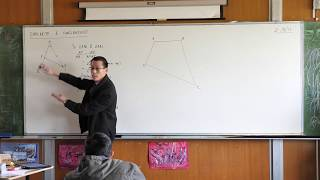10:56
Midpoints & Parallel Lines in Polygons (1 of 3: Any triangle)
2y8m ago06:03
Locus of a Parabola (2 of 3: Deriving the basic equation)
2y8m ago10:14
Right-Angled Triangle Trigonometry (2 of 2: Example basic questions)
2y8m ago08:48
Right-Angled Triangle Trigonometry (1 of 2: Reviewing the ratios)
2y8m ago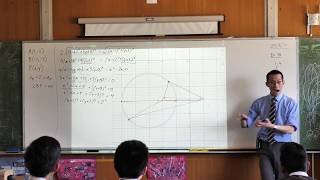11:37
Locus: A Surprising Definition of a Familiar Shape
2y8m ago06:38
Introduction to Locus (3 of 3: Determining locus equations)
2y8m ago09:59
Introduction to Locus (2 of 3: Using coordinates to assist)
2y8m ago11:40
Introduction to Locus (1 of 3: Exploring shapes through geometric rules)
2y8m ago09:56
Quadratic Identities (2 of 2: Using three arbitrary values)
2y8m ago07:59
Quadratic Identities (1 of 2: By comparison of coefficients)
2y8m ago08:19
Sum & Product of Roots (5 of 5: Forming a quadratic with given roots)
2y8m ago07:32
Sum & Product of Roots (4 of 5: Working with related roots)
2y8m ago10:30
Sum & Product of Roots (3 of 5: Basic example questions)
2y8m ago09:55
Sum & Product of Roots (2 of 5: Comparing coefficients)
2y8m ago11:39
Sum & Product of Roots (1 of 5: Introduction to Viete's Results)
2y8m ago06:24
Definite & Indefinite Quadratics (2 of 2: Example questions)
2y8m ago06:07
Definite & Indefinite Quadratics (1 of 2: Using the discriminant)
2y8m ago06:57
Solving a Quartic Equation with Quadratic Techniques
2y8m ago09:26
Mathematical Induction Divisibility Proof (3 of 3: Proving the k+1 case)
2y8m ago04:42
Mathematical Induction Divisibility Proof (2 of 3: Test and assumption)
2y8m ago11:00
Mathematical Induction Divisibility Proof (1 of 3: What is inductive logic?)
2y8m ago04:31
Max/Min Question: Cutting a Wire in Two (2 of 2: Finding the minimum)
2y8m ago10:08
Max/Min Question: Cutting a Wire in Two (1 of 2: Setting up the equations)
2y8m ago06:01
The Power Curves (2 of 2: Considering higher powers)
2y8m ago07:20
The Power Curves (1 of 2: Investigating through tables of values)
2y8m ago05:47
Maximum/Minimum with Quadratics (2 of 2: Completing the square)
2y8m ago08:30
Maximum/Minimum with Quadratics (1 of 2: Axis of symmetry)
2y9m ago10:08
Graphs of Logarithms (3 of 3: Visualising the shape)
2y9m ago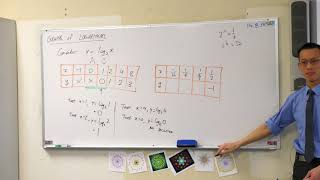09:16
Graphs of Logarithms (2 of 3: Investigating near x=0)
2y9m ago07:59
Graphs of Logarithms (1 of 3: Considering integer values)
2y9m ago08:37
Equations Reducible to Quadratics (Changing Logarithm Bases)
2y9m ago05:26
Solving Equations with Radical Functions (2 of 2: Final solution)
2y9m ago10:58
Solving Equations with Radical Functions (1 of 2: Initial steps & discussion)
2y9m ago10:00
Equation of a Parabola - Vertex Form (Completing the square)
2y9m ago06:10
Y10 Review Questions (Solving quadratic equations)
2y9m ago08:34
Proof by Contradiction (1 of 2: How does it work?)
2y9m ago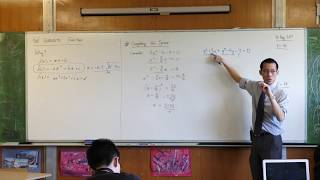06:58
Introduction to Quadratic Theory (2 of 2: Completing the square)
2y9m ago06:4506:4207:3413:20